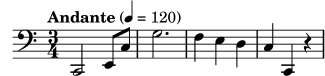### 2.2.1 Simple notation

LilyPond adds some notation elements automatically. In the next example, we have only specified four pitches, but LilyPond has added a clef, a time signature, and rhythms.

```{
c' e' g' e'
}
```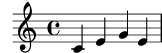This behavior may be altered, but in most cases these automatic values are useful.

#### Pitches

Music Glossary: pitch, interval, scale, middle C, octave, accidental.

LilyPond uses lowercase letters for pitches. Note names in all examples in this section use the Dutch naming system (white piano keys are c, d, e, f, g, a, b). However, LilyPond supports many different naming schemes like ‘English’ or ‘Fixed-Do’ (do, re, mi, …). See Note names in other languages. The letters ‘c’ through ‘b’ denote pitches in the ‘small octave’ below middle C. Added ‘'’ or ‘,’ suffixes indicate higher or lower octaves. Here is a scale starting on middle C, and an arpeggio:

```{ c' d' e' f' g' a' b' c''
g c' e' g' c'' e'' g'' c''' }
```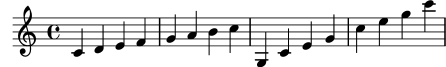The easiest way to enter notes is by using `\relative` mode. In this mode, the octave is chosen automatically by assuming that the following note is always to be placed closest to the previous note, i.e., it is to be placed in the octave that is within three staff spaces of the previous note. We begin by entering the most elementary piece of music, a scale, in which every note is within just one staff space of the previous note.

```\relative {
c' d e f
g a b c
}
```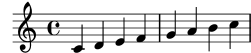The initial note is middle C, denoted by `c'`. Each successive note is placed closest to the previous note – in other words, the first ‘c’ is the closest C to middle C. This is followed by the closest D to the previous note. We can create melodies that have larger intervals, still using only relative mode:

```\relative {
d' f a g
c b f d
}
```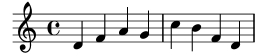In the previous example, the first note – the `d'` with one ‘'’ mark – is the D in the octave starting from middle C and going up to B.

By adding (or removing) quotes (‘'’) or commas (‘,’) from the first note, we can change the starting octave:

```\relative {
e'' c a c
}
```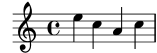Relative mode can be confusing initially, but is the easiest way to enter most melodies. Let us see how this relative calculation works in practice. Starting from a B, which is on the middle line in a treble clef, you can reach a C, D and E within 3 staff spaces going up, and an A, G and F within 3 staff spaces going down. So if the note following a B is a C, D or E it is assumed to be above the B, and an A, G or F is assumed to be below.

```\relative {
b' c % c is 1 staff space up, so is the c above
b d  % d is 2 up or 5 down, so is the d above
b e  % e is 3 up or 4 down, so is the e above
b a  % a is 6 up or 1 down, so is the a below
b g  % g is 5 up or 2 down, so is the g below
b f  % f is 4 up or 3 down, so is the f below
}
```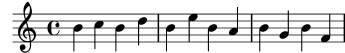Exactly the same happens even when any of these notes are sharpened or flattened. Accidentals are totally ignored in the calculation of the relative position. Precisely the same staff space counting is done from a note at any other position on the staff.

To add intervals that are larger than three staff spaces, we can raise the octave by adding a single quote ‘'’ (or apostrophe) to the note name. We can lower the octave by adding a comma ‘,’ to the note name.

```\relative {
a' a, c' f,
g g'' a,, f'
}
```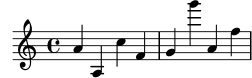To change a note by two (or more!) octaves, we use multiple `''` or `,,` – but be careful that you use two single quotes `''` and not one double quote ‘"’!

#### Durations (rhythms)

Music Glossary: beam, duration, whole note, half note, quarter note, dotted note.

The duration of a note is specified by a number after the note name: ‘1’ for a whole note, ‘2’ for a half note, ‘4’ for a quarter note and so on. Beams are added automatically.

If you do not specify a duration, the previous duration is used for the next note. The duration of the first note defaults to a quarter note.

```\relative {
a'1
a2 a4 a8 a
a16 a a a a32 a a a a64 a a a a a a a a2
}
```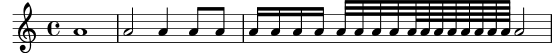To create dotted notes, add a dot (‘.’) to the duration number. The duration of a dotted note must be stated explicitly (i.e., with a number).

```\relative {
a'4 a a4. a8
a8. a16 a a8. a8 a4.
}
```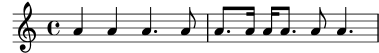#### Rests

Music Glossary: rest.

A rest is entered just like a note with the name ‘r’.

```\relative {
a'4 r r2
r8 a r4 r4. r8
}
```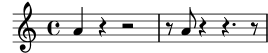#### Time signature

Music Glossary: time signature.

The time signature can be set with the `\time` command:

```\relative {
\time 3/4
a'4 a a
\time 6/8
a4. a
\time 4/4
a4 a a a
}
```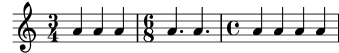#### Tempo marks

Music Glossary: tempo indication, metronome.

The tempo indication and metronome mark can be set with the `\tempo` command:

```\relative {
\time 3/4
\tempo "Andante"
a'4 a a
\time 6/8
\tempo 4. = 96
a4. a
\time 4/4
\tempo  "Presto" 4 = 120
a4 a a a
}
```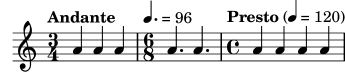#### Clef

Music Glossary: clef.

The clef can be set using the `\clef` command:

```\relative {
\clef treble
c'1
\clef alto
c1
\clef tenor
c1
\clef bass
c1
}
```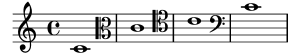#### All together

Here is a small example showing all these elements together:

```\relative {
\clef bass
\time 3/4
\tempo "Andante" 4 = 120
c,2 e8 c'
g'2.
f4 e d
c4 c, r
}
```